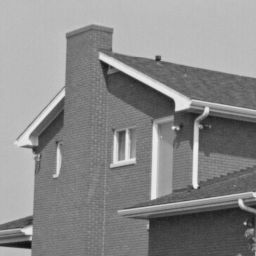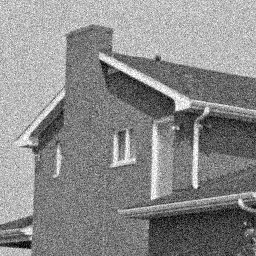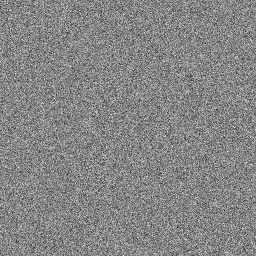图像处理两张图相减_两张相同的图像相减为什么得到的不是一张黑色的图 - CSDN
• 看完了数字图像处理后，从头开始使用opencv进行相关内容的实现，使用的环境是VS2013+OpenCV2.4.9 1.图像的加运算 加运算就是两幅图像对应像素的灰度值或彩色分量进行相加。主要有种用途，一种是消除图像的随机噪声...
看完了数字图像处理后，从头开始使用opencv进行相关内容的实现，使用的环境是VS2013+OpenCV2.4.9
1.图像的加运算
加运算就是两幅图像对应像素的灰度值或彩色分量进行相加。主要有两种用途，一种是消除图像的随机噪声，主要做是讲同一场景的图像进行相加后再取平均；另一种是用来做特效，把多幅图像叠加在一起，再进一步进行处理。
对于灰度图像，因为只有单通道，所以直接进行相应位置的像素加法即可，对于彩色图像，则应该将对应的颜色的分量分别进行相加。
通常来将，两幅或多幅相加的图像的大小和尺寸应该相同。

经过师兄提醒，在这里补充一下，在OpenCV中除了上述使用函数的方法外，还可以直接运用运算符进行操作，例如在求两个图像的和的时候，可以直接写为

result1 = (img1 + img2) / 2;在进行二值化时，再将图像灰度化后，可以直接使用类似语句

result = (img1 > 30);

在实际的使用中，灵活的运用运算符进行图像的操作可以带来很大的方便。

PS：再次感谢师兄的提醒 :D

//本程序实现将两张尺寸相同的图像进行相加
//在对像素进行操作时，使用的时迭代器的方法
//2017-02-18

#include <iostream>
#include <opencv2\core\core.hpp>
#include <opencv2\highgui\highgui.hpp>

using namespace cv;
using namespace std;

int main()
{
Mat img1, img2, result1;
result1 = img1.clone();
Mat_<Vec3b>::iterator it = result1.begin<Vec3b>();	//result1初始位置的迭代器
Mat_<Vec3b>::iterator itend = result1.end<Vec3b>();	//result1终止位置的迭代器
Mat_<Vec3b>::iterator it1 = img1.begin<Vec3b>();   //img1初始迭代器
Mat_<Vec3b>::iterator it2 = img2.begin<Vec3b>();	//img2初始迭代器
//进行遍历
for (; it != itend; it++)
{
(*it) = ((*it1) + (*it2)) /2;
(*it) = ((*it1) + (*it2)) /2;
(*it) = ((*it1) + (*it2)) /2;
it1++;
it2++;
}
namedWindow("原图1",1);
imshow("原图1", img1);
namedWindow("原图2", 0);
imshow("原图2", img2);
namedWindow("相加后的图像",0);
imshow("相加后的图像", result1);
Mat result2 = result1.clone();
waitKey();
return 0;
}

运行结果为：2.图像相减
减法运算就是两幅图像见对象像素的灰度值或彩色分量进行相减，它可以用于目标检测，程序实现还是使用两种方法。
以下为程序部分

//本程序实现两个尺寸相同的图像进行相减的操作

#include <iostream>
#include <opencv2\core\core.hpp>
#include <opencv2\highgui\highgui.hpp>

using namespace std;
using namespace cv;
uchar toZero(uchar a);//置零函数，小于零则为0
int main()
{
Mat imag1, imag2, result1, result2;
result1 = imag1.clone();
result2 = imag2.clone();

int rowNumber = result1.rows;
int colNumber = result1.cols;
for (int i = 0; i < rowNumber; i++)
{
for (int j = 0; j < colNumber; j++)
{
result1.at<Vec3b>(i, j) = toZero(imag1.at<Vec3b>(i, j) - imag2.at<Vec3b>(i, j));
result1.at<Vec3b>(i, j) = toZero(imag1.at<Vec3b>(i, j) - imag2.at<Vec3b>(i, j));
result1.at<Vec3b>(i, j) = toZero(imag1.at<Vec3b>(i, j) - imag2.at<Vec3b>(i, j));
}
}
imshow("原图1", imag1);
imshow("原图2", imag2);
imshow("result1", result1);
waitKey();
return 0;
}

uchar toZero(uchar a)
{
if (a < 0)
return 0;
else
return a;
}

运行结果为：result1貌似有问题，需要进一步进行检查。

3.图像相乘
图像的乘法运算就是将两幅图像对应的灰度值或彩色分量进行相乘。
乘运算的主要作用是抑制图像的某些区域，掩膜值置为1，否则置为0。乘运算有时也被用来实现卷积或相关的运算。
以下为相关程序代码。

//图像的乘运算。
//将两幅图像对应的灰度值或彩色分量进行相乘。

#include <iostream>
#include <opencv2\core\core.hpp>
#include <opencv2\highgui\highgui.hpp>

using namespace std;
using namespace cv;

int main()
{
Mat imag1, imag2, result;
result = imag1.clone();
int rowNumber = result.rows;
int colNumber = result.cols;
for (int i = 0; i < rowNumber; i++)
{
for (int j = 0; j < colNumber; j++)
{
result.at<Vec3b>(i, j) = (imag1.at<Vec3b>(i, j) * imag2.at<Vec3b>(i, j)) % 256;
result.at<Vec3b>(i, j) = (imag1.at<Vec3b>(i, j) * imag2.at<Vec3b>(i, j)) % 256;
result.at<Vec3b>(i, j) = (imag1.at<Vec3b>(i, j) * imag2.at<Vec3b>(i, j)) % 256;
}
}
imshow("原图1", imag1);
imshow("原图2", imag2);
imshow("相乘后的图像", result);
waitKey();
return 0;
}实验的结果如下：4.图像相除
图像除运算就是两幅图像对应像素的灰度值或彩色分量进行相除。
简单的出运算可以用于改变图像的灰度级

以下为代码部分

//图像的除法运算
//就是讲两幅图像的对应像素的灰度值或彩色分量进行相除。
//简单的除运算可以用来改变图像的灰度级。

#include <iostream>
#include <opencv2\core\core.hpp>
#include <opencv2\highgui\highgui.hpp>

using namespace std;
using namespace cv;

int main()
{
Mat imag1, imag2, result;
result = imag1.clone();

int rowNumber = result.rows;
int colNumber = result.cols;
for (int i = 0; i < rowNumber; i++)
{
for (int j = 0; j < colNumber; j++)
{
result.at<Vec3b>(i, j) = (imag1.at<Vec3b>(i, j) * 1.0) / imag2.at<Vec3b>(i, j);
result.at<Vec3b>(i, j) = (imag1.at<Vec3b>(i, j) * 1.0) / imag2.at<Vec3b>(i, j);
result.at<Vec3b>(i, j) = (imag1.at<Vec3b>(i, j) * 1.0) / imag2.at<Vec3b>(i, j);
}
}
imshow("原图1", imag1);
imshow("原图2", imag2);
imshow("相除后的图像", result);
waitKey();
return 0;
}


展开全文opencv
• 关键函数cvAbsDiff() ,代码如下： #include "cv.h" #include "highgui.h" #include "cxcore.h" int main(int argc,char** argv) {cvNamedWindow("a",0);  IplImage* img=cvLoadImage(... cvShowImage(

关键函数cvAbsDiff()

,代码如下：

#include "cv.h"
#include "highgui.h"
#include "cxcore.h"

int main(int argc,char** argv)
{cvNamedWindow("a",0);
cvShowImage("a",img);

cvNamedWindow("b",0);
cvShowImage("b",img1);

IplImage* diff=cvCreateImage(cvGetSize(img),img->depth,img->nChannels);
cvAbsDiff(img,img1,diff);
cvNamedWindow("r",0);
cvShowImage("r",diff);

while (1)
{if (cvWaitKey(100)==27) break;
}

cvDestroyWindow("a");
cvDestroyWindow("b");
cvReleaseImage(&img);
cvReleaseImage(&img1);
cvReleaseImage(&diff);
return 0;
}

展开全文• 首先保证两幅图片大小一样，然后直接减，我也在车型识别，可以探讨。 i=imread('01.bmp'); >> j=imread('02.bmp'); >> i1=rgb2gray(i); >> j1=rgb2gray(j);%%%%%%%%%%%%%%%%%%%灰度化 >> s=imsubtract(j1,i1); ...

首先保证两幅图片大小一样，然后直接减，我也在车型识别，可以探讨。
>> i1=rgb2gray(i);
>> j1=rgb2gray(j);%%%%%%%%%%%%%%%%%%%灰度化
>> s=imsubtract(j1,i1);
>> imshow(s)

展开全文matlab 图片
• 这是因为当我们使用imread函数直接去加载图像时，读取的图像格式是 CV_8UC3 即为uint8 是unsigned 的，因此在图像相减过程中会自动将像素值限定在[0,255]范围内。假设img1中像素值为（12,3,255），img2像素值为（13,...
这是因为当我们使用imread函数直接去加载图像时，读取的图像格式是 CV_8UC3 即为uint8 是unsigned 的，因此在图像相减过程中会自动将像素值限定在[0,255]范围内。假设img1中像素值为（12,3,255），img2像素值为（13,4,23），则img1-img2的像素值为（255,255,232），但是我们实际上是需要（-1，-1, 232）。为了解决这个问题，我们可以在读取图片时，将图片的格式改为int32的形式，这样就可以让负数不直接变为0。下面对应两种相减方式，以及其对应的效果。为了更好的体现差值图像的效果，我们对差值图像还做了归一化的操作。img1                                                       img2

一、直接相减，及其差值图像

def grey_scale(img_gray ):
//归一化函数
rows, cols = img_gray.shape
flat_gray = img_gray.reshape((cols * rows,)).tolist()
A = min(flat_gray)
B = max(flat_gray)
print('A = %d,B = %d' % (A, B))
output = np.uint8(255 / (B - A) * (img_gray - A) + 0.5)
return output

img=img_1-img_2
result=grey_scale(img) //图片归一化
cv2.imwrite("C:\\Users\\ASUS\\Desktop\\9c.jpg",result)二、读取时改变图像类型，再相减操作

def grey_scale(img_gray ):
//归一化函数
rows, cols = img_gray.shape
flat_gray = img_gray.reshape((cols * rows,)).tolist()
A = min(flat_gray)
B = max(flat_gray)
print('A = %d,B = %d' % (A, B))
output = np.uint8(255 / (B - A) * (img_gray - A) + 0.5)
return output

img=img_1-img_2
result=grey_scale(img) //归一化操作
cv2.imwrite("C:\\Users\\ASUS\\Desktop\\9c.jpg",result)差值图像

展开全文图像处理 归一化 图像去噪
• 假设f(x,y),g(x,y)分别为两幅已知的图像图像的线性操作就是直接在每个位置的像素上做运算(两幅图像的尺寸需保持一致)，最终得到的图像s(x,y)，可能会出现以下问题: 1）会出现不在 [ 0-255 ] 之间的像素点存在，最...数字图像 加减乘除
• opencv 图像帧差法（图像相减） 代码 /* 说明这种方法经过调试在vc下是可以的，但在codeblocks下不可以，问题出在height变量上，如果height的数值改的小些 则可以，但有部分图像未得到处理，而且使用cvSet()...opencv
• 视频图像动态检测原理实验实验原理，输入两幅图像s1 / s2 ，然后将两张图片相减，如果变换的像素超过一定阈值则说明图像中有运动物体。实验1： 直接使用opencv提供的图像减法功能：Cv2.imread默认读取的是全彩图片...python opencv
• 数字图像处理:如何通过背景相减提取物体轮廓？ 因为在数学建模比赛中需要用到数字图像处理，从0开始学习，自己把期间做的笔记整理下来。不积跬步无以至千里。 一、理论 1、图像的数字化采集 图像采样就是按照图像...数字图像处理 MATLAB
• 涉及：图片读取，图片保存，感兴趣区域选取，图像相减 找出下面两张图之间的八个不同 原： 代码： #include<iostream> #include<opencv2/opencv.hpp> using namespace std; using namespace cv; ...图像处理
• 由于最近在做图像处理的项目，有时候需要快速的知道图像的最大像素值和最小像素值是多少，或者图像的最大最小像素的坐标在哪里。需要快速的得到RGB图像中的R、G、B当中的某个通道。需要把RGB图像转成YUV数据存储。...
• 第一种： 关键函数cvAbsDiff() 代码如下： #include "cv.h" #include "highgui.h" #include "cxcore.h" ...int main(int argc,char** argv) ...IplImage* img=cvLoadImage("11.jpg");...cvShowImage("a",im...
• 简单的处理：通过将图片每一个像素的RGB值...将两张图片同一位置的像素相减小于阀值，（颜色阈值:图像的转换是比较像素的过程,在比较两个像素时,如果RGB的颜色值 的差异小于颜色阈值,则可以认为这两个像素是相同...
• 1.有一个小游戏，就给出两张内容几乎差不多全部相同的图像，让大家在最快的时间内找出两个图像中有几处不同地方，我这里试着用OpenCV实现这个功能。 2.我的编程环境是Windows 7 64位，IDE是VS2015，配置了OpenCV3.3...OpenCV3 计算机视觉 帧差法 大家来找茬
• 来自：shiter编写程序的艺术 ...对计算图像相似度的方法，本文做了如下总结，主要有三种办法：1.PSNR峰值信噪比PSNR（Peak Signal to Noise Ratio），一种全参考的图像质量评价指标。简介：https://en.wikipedia.opencv 图形图像处理
• 最近做的DEM图像处理的小程序，遇到异常，整理如下： （1）栅格求差，可以运行，但是结果很诡异，完全不是求差。多次检查栅格计算的脚本，以为是栅格计算的语句出错。多次检查运行语句，多次试验，结果还是还是很...
• 环境准备： Python 2.7 or Python 3 ...比较两张图片是不是一个图片 如何运行 python file.py 复制代码代码如下 #!C:/Python27 & Python3 #coding=utf-8 # 1. pip install pillow # 2. pip install py...
...